Win up to 100% scholarship on Aakash BYJU'S JEE/NEET courses with ABNAT Win up to 100% scholarship on Aakash BYJU'S JEE/NEET courses with ABNAT

# Venn Diagram in Set Theory

Venn diagrams usually represent a set. It is the pictorial relationship of two or more sets where everything, apart from the elements of the sets, is called a universal set. Usually, universal sets are represented by rectangular regions, disjoint sets by disjoint circles and intersecting sets by intersecting circles. By universal sets, we mean the maximum number of possible points that can be included in any set.

## Representation of Sets with Venn Diagrams

Case 1: When universal sets and a normal set have been given.

Let U be the universal set representing the sets of all natural numbers, and let A ⊆ U for A = 1,2,3,4,5

Then, by a Venn diagram, we can show as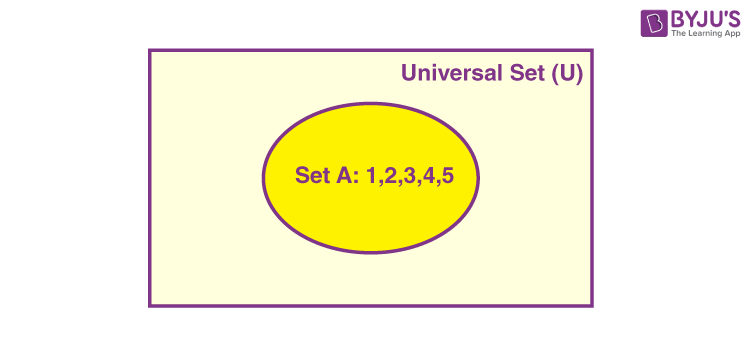Case 2: When two intersecting subsets of U are given.

To represent two intersecting subsets, A and B of U, we draw two circles, and the intersecting regions will be shown as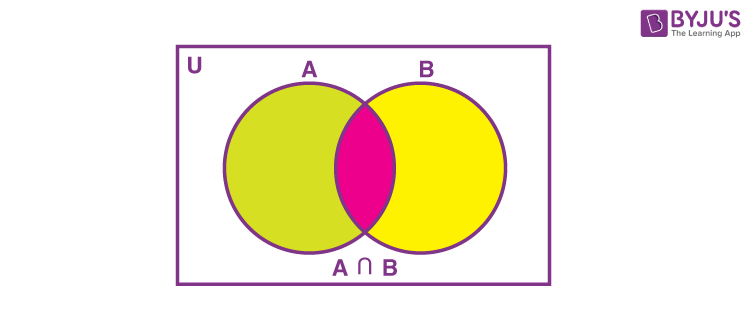Case 3: When two disjoint sets are given.

To draw two disjoint sets, we normally draw two circles which will not intersect each other within a rectangle.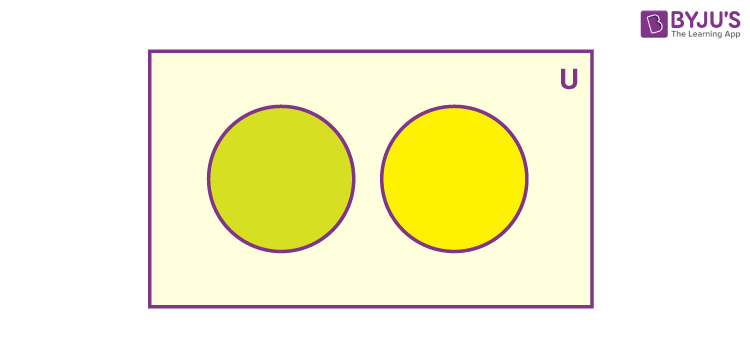Case 4: When B ⊆ A ⊆ U

In this case, we draw two concentric circles within a rectangular region.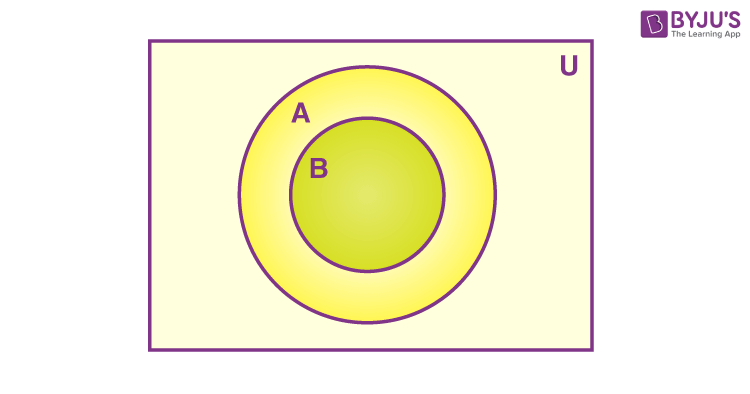Case 5: Complement of a set.

Let U be the set of natural numbers, and let A = {1, 2, 3}. Then, the complement of set A is given by

A’ = U – A = {1 2, 3, 4, 5, 6,….} – {1, 2, 3} = {4, 5, 6,…}

Then, the complement of A can be shown as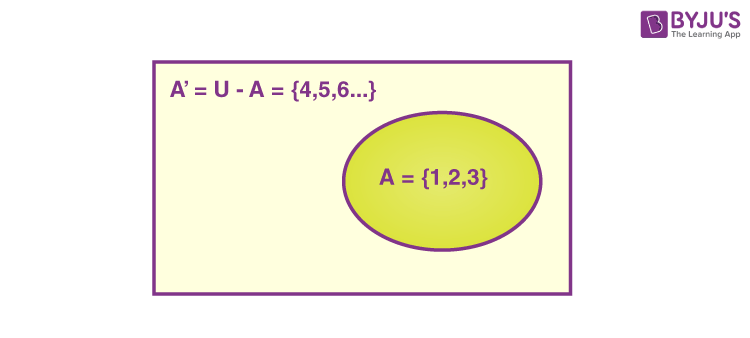## Solved Examples

Example 1: Consider the relations.

a) A – B = A – (A ∩ B)

b) A = (A ∩ B) ∪ (A – B)

c) A – (B ∩ C) = (A – B) ∪ (A – C)

Which of these options is/are correct?

1. a and c
2. b only
3. b and c
4. a and b

a) A – B = A – (A ∩ B) is correct.

b) A = (A ∩ B) ∪ (A – B) is correct.

c) A – (B ∩ C) = (A – B) ∪ (A – C) is false.

So, (a) and (b) are true.

Option (d) is the correct answer.

Example 2: Which is the simplified representation of (A’∩ B’∩ C) ∪ (B ∩ C) ∪ (A ∩ C)?

a) A

b) B

c) C

d) X ∩ (A ∪ B ∪ C)

(A’∩ B’∩ C) = only C

(A’∩ B’ ∩ C) ∪ (B ∩ C) ∪ (A ∩ C) = C (by Venn Diagram)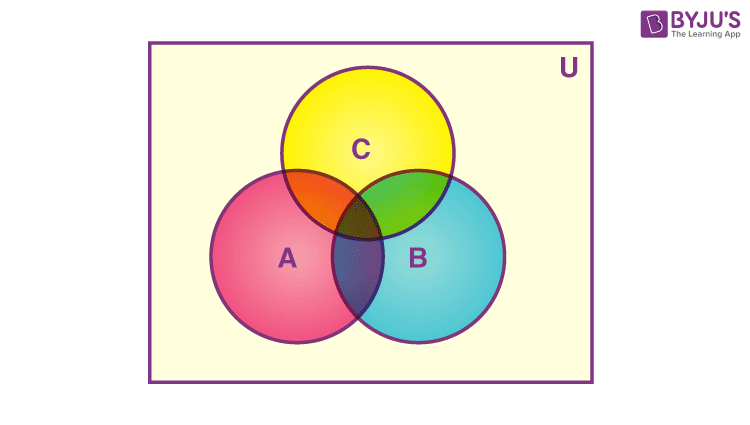Example 3: In a class of 25 students, 12 students took singing, 11 took dancing, and 15 took writing; 4 took both singing and dancing; 9 took dancing and writing; 5 took writing and singing. 3 took all three. Find the number of students who took

1. Singing only
2. Dancing only
3. Writing only
4. Singing and dancing but not writing
5. Writing and dancing but not singing
6. At least one of the three
7. None of the three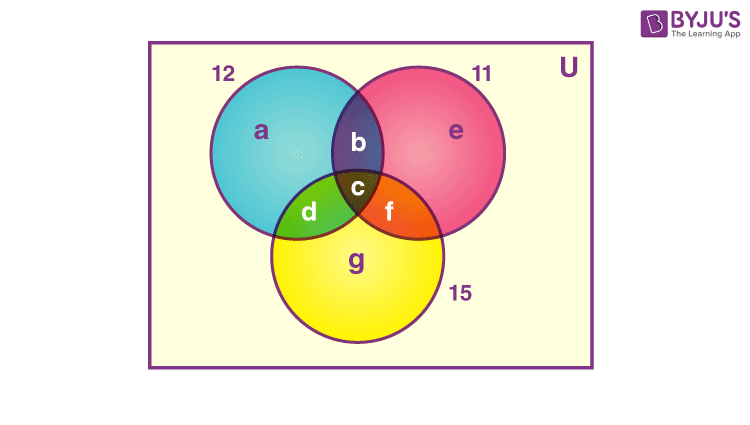Let the number of students in the respective regions be represented by a, b, c, d, e, f, and g, as shown above.

As per the question given, the data are

a + b + c + d = 12

b + c + e + f = 11

c + d + f + g = 15

b + c = 4

c + f = 9

c + d = 5

c = 3

By following these equations, we get

c = 3; f = 6; d = 2; b = 1

Now,

c + d + f + g = 15 ⇒ 3 + 2 + 6 + g = 15 ⇒ g = 4

b + c + e + f = 11 ⇒ 1 + 3 + e + 6 = 11 ⇒ e = 1

a + b + c + d = 12 ⇒ a + 1 + 3 + 2 = 12 ⇒ a = 6

So, we have

Number of students who took singing only (a) = 6

Number of students who took dancing only (e) = 1

Number of students who took writing only (g) = 4

Number of students who took singing and dancing but not writing = 1

Number of students who took singing and writing but not dancing = 2

Number of students who took none = (25 – 23) = 2

Number of students who took only one = 6 + 1 + 4 = 11

Number of students who took at least one of the given options = 6 + 1 + 3 + 2 + 1 + 6 + 4 = 23

## Solving JEE Advanced PYQ – Venn Diagram ProblemsQ1

### What do you mean by a Venn Diagram?

A Venn Diagram is a pictorial representation of all possible relations of different sets.

Q2

### Who invented the Venn Diagram?

John Venn is the inventor of the Venn Diagram.

Q3

### What do you mean by the union of two sets?

The union of two sets is the set containing all the elements of the given sets.

Q4

### How can we represent two disjoint sets in a Venn Diagram?

To represent two disjoint sets, we draw two circles which will not intersect each other within a rectangle.